# 天然黄铁矿对RhB和SRB的吸附特性比较研究Comparison Study of Adsorption Characteristics of Natural Pyrite to RhB and SRB

DOI: 10.12677/AEP.2019.93054, PDF, HTML, XML, 下载: 309  浏览: 467  国家自然科学基金支持

1. 引言

2. 实验部分

2.1. 主要仪器及试剂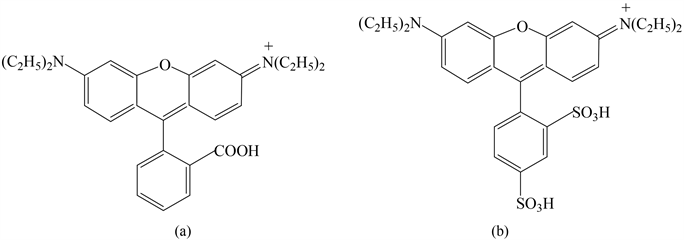Figure 1. Structure of RhB (a) and SRB (b)

2.2. 实验方法

2.2.1. 标准曲线的绘制

SRB标准曲线的绘制方法同RhB，检测波长为565 nm。

2.2.2. 吸附实验

$去除率\left(%\right)=\frac{{c}_{0}-{c}_{e}}{{c}_{0}}×100%$ (1)

${q}_{t}=\frac{\left({c}_{0}-{c}_{t}\right)v}{m}$ (2)

$\mathrm{log}\left({q}_{e}-{q}_{t}\right)=\mathrm{log}{q}_{e}-\frac{{k}_{1}}{2.303}t$ (3)

$\frac{t}{{q}_{t}}=\frac{1}{{k}_{2}{q}_{e}{}^{2}}+\frac{t}{{q}_{e}}$ (4)

$\frac{{c}_{e}}{{q}_{e}}=\frac{1}{{k}_{{}_{L}}}+\frac{{a}_{L}}{{k}_{L}}{c}_{e}$ (5)

$\mathrm{ln}{q}_{e}=\mathrm{ln}{k}_{F}+\frac{1}{{n}_{F}}\mathrm{ln}{c}_{e}$ (6)

ce (mg/L)为吸附达到平衡时溶液中剩余的底物的浓度；qe (mg/g)是吸附达到平衡时底物被吸附的浓度；kL (L/g)和aL (L/mg)为Langmuir吸附等温线常数；kF为Freundlich吸附等温线常数(L/mg)；1/nF是非均质性参数。

$\mathrm{ln}{K}_{d}=\frac{\Delta {S}^{0}}{R}-\frac{\Delta {H}^{0}}{RT}$ (7)

$\Delta {G}^{0}=\Delta {H}^{0}-T\Delta {S}^{0}$ (8)

3. 结果与讨论

3.1. 标准曲线的绘制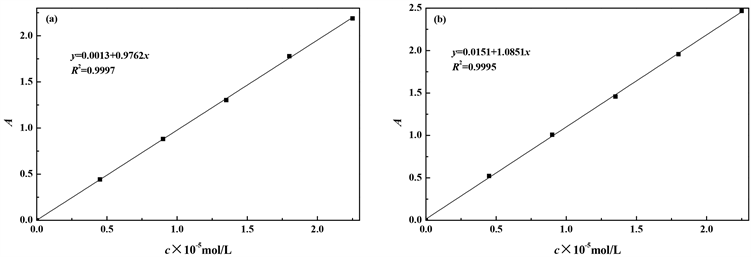Figure 2. Standard curves of RhB (a) and SRB (b)

3.2. 吸附动力学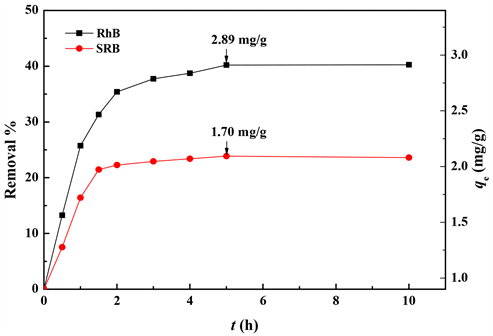T = 20℃ ± 1℃；v = 50 mL；m = 25 mg；c0 = 1.5 × 10−5 mol/L；pH = 7 ± 0.1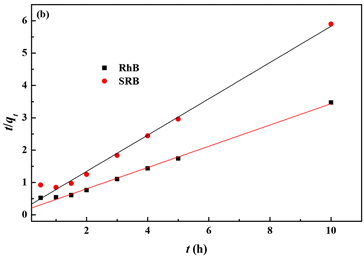Figure 4. Plot of pseudo-first-(a) and pseudo-second-order rate (b) for the adsorption of RhB and SRB on pyrite powderTable 1. Comparison of pseudo-first- and pseudo-second-order model parameters

3.3. 吸附等温线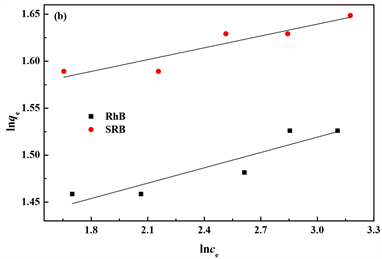Figure 5. Adsorption isotherm of RhB and SRB on pyrite powderTable 2. Parameters for Langmuir and Freundlich sorption isotherms

3.4. 热力学研究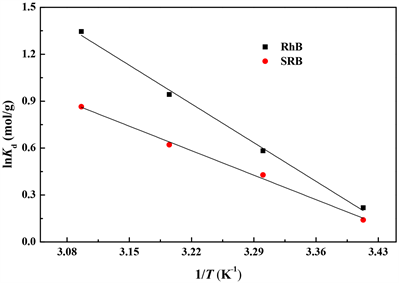Figure 6. Effect of temperature on adsorption of RhB and SRB by pyrite powderTable 3. Thermodynamic parameters at different temperatures

3.5. 溶液pH对吸附过程的影响Figure 7. Adsorption of RhB and SRB by pyrite power at different pH

4. 黄铁矿粉末再生处理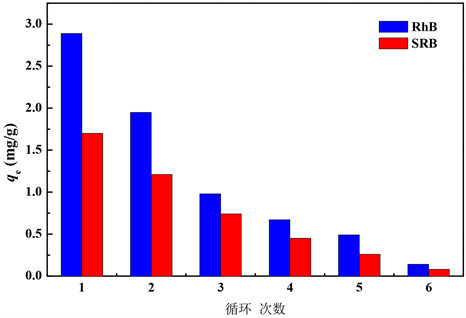Figure 8. Cyclic adsorption of RhB and SRB on pyrite powder

5. 结论

NOTES

*通讯作者。

  Zhang, P., Yuan, S. and Liao, P. (2016) Mechanisms of Hydroxyl Radical Production from Abiotic Oxidation of Pyrite under Acidic Conditions. Geochimica et Cosmochimica Acta, 172, 444-457. https://doi.org/10.1016/j.gca.2015.10.015  Kong, L., Hu, X. and He, M. (2015) Mechanisms of Sb(III) Oxidation by Pyrite-Induced Hydroxyl Radicals and Hydrogen Peroxide. Environmental Science & Technology, 49, 3499-3505. https://doi.org/10.1021/es505584r  Lin, Y.T. and Huang, C.P. (2008) Reduction of Chromium(VI) by Pyrite in Dilute Aqueous Solutions. Separation and Purification Technology, 63, 191-199. https://doi.org/10.1016/j.seppur.2008.05.001  Pham, H.T., Kitsuneduka, M., Hara, J., Suto, K. and Inoue, C. (2008) Tri-chloroethylene Transformation by Natural Mineral Pyrite: The Deciding Role of Oxygen. Environmental Science & Technology, 42, 7470-7475. https://doi.org/10.1021/es801310y  Bae, S., Kim, D. and Lee, W. (2013) Degradation of Diclofenac by Pyrite Catalyzed Fenton Oxidation. Applied Catalysis B: Environmental, 134-135, 93-102. https://doi.org/10.1016/j.apcatb.2012.12.031  Zhang, Y., Zhang, K., Dai, C. and Zhou, X. (2014) An Enhanced Fenton Reaction Catalyzed by Natural Heterogeneous Pyrite for Nitrobenzene Degradation in an Aqueous Solution. Chemical Engineering Journal, 244, 438-445. https://doi.org/10.1016/j.cej.2014.01.088  Zhang, Y., Tran, H.P., Hussain, I., Zhong, Y. and Huang, S. (2015) Degradation of p-Chloroaniline by Pyrite in Aqueous Solutions. Chemical Engineering Journal, 279, 396-401. https://doi.org/10.1016/j.cej.2015.03.016  冯勇, 吴德礼, 马鲁铭. 黄铁矿催化类Fenton反应处理阳离子红X-GRL废水[J]. 中国环境科学, 2012, 32(6): 1011-1017.  张菁, 李睿华, 李杰, 刘波. 天然黄铁矿的除磷性能[J]. 环境工程学报, 2013, 7(10): 3856-3860.  王丹, 刘洪芳, 钱天伟. 天然黄铁矿对水溶液中硒酸根的吸附性能研究[J]. 环境污染与防治, 2014, 36(3): 30-34.  万晶晶, 郭楚玲, 涂志红, 党志. 黄铁矿对水中六价铬的吸附去除[J]. 化工环保, 2016, 36(5): 506-510.  蔡宽, 熊世威, 张欣欣, 李瑞萍, 黄应平. 天然黄铁矿对阳离子有机染料RhB吸附特性研究[J]. 岩石矿物学杂志, 2014, 33(2): 370-376.  Li, L., Liu, S. and Zhu, T. (2010) Application of Activated Carbon Derived from Scrap Tires for Adsorption of Rhodamine B. Journal of Environmental Sciences, 22, 1273-1280. https://doi.org/10.1016/S1001-0742(09)60250-3